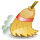ReNamer:Pascal Script:Basic Conversion RoutinesThis article needs to be cleaned up!

Basic Conversion Routines or How to serialize files

In this chapter we will take a look on serialization. All we need to serialize filenames is an integer variable and a way to convert the number into a string. This type of convertion is done by IntToStr function. Convertion is necessary as FileName is a string and anything we want to add to a FileName must be also a string.

In the following script we will add a serialized number in front of the filename.

var
i : Integer;

begin
FileName:=IntToStr(i)+'_'+FileName;
i:=i+1;
end.

This script always serialize from 0 to "infinity". It’s because all the variables are set to their default values when you press Preview. And the default value of integers is 0.

Would you mind trying to rewrite this script so it would start to serialize from 1? It’s enough to swap the main two lines of code.

i:=i+1;
FileName:=IntToStr(i)+’_’+FileName;

Now i is incremented from default 0 to 1 before the first filename is changed.

For now we can serialize from 0 or 1. How to start from a different number will be covered in the next chapter.

And what if we have filenames that starts from two-digit random number and we want to increase these numbers eg. by 2?

We need to extract the number from the FileName string, convert it to integer, increase by 2 and then convert back to string. It means we would need the StrToInt function which is the complete opposition to already known IntToStr.

var
i : Integer;
Number : String;

begin
Number:=Copy(FileName, 1, 2);
i:=StrToInt(Number);
if i >= 0 then
begin
i:=i+2;
FileName:=IntToStr(i)+WideCopy(FileName, 3, Length(FileName)-2);
end;
end.

If the string passed to StrToInt function cannot be converted into integer, function will return -1.

If that doesn’t suit us (cause we want to convert ’-1’ strings into integers as well and we still want to be warned when the convertion fails) we may use StrToIntDef function that apart from the string takes the default integer value as a parameter and returns that value on failure. So we could use

i:=StrToIntDef(Number, -123);
if i <> -123 then...

in our code.